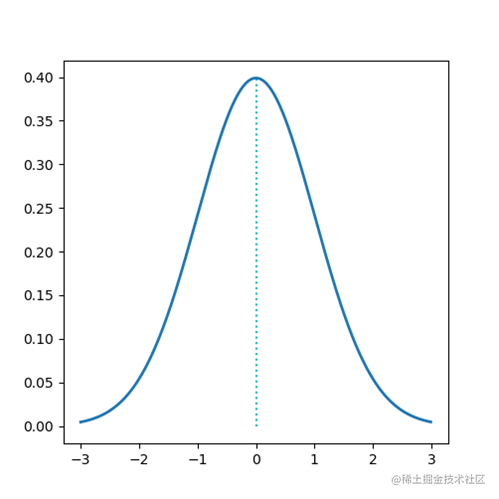# 遗传算法中常见遗传算子

## 常见选择算子

### 轮盘选择（roulette wheel selection）

A87%
B1210%
C2723%
D43%
E4539%
F1715%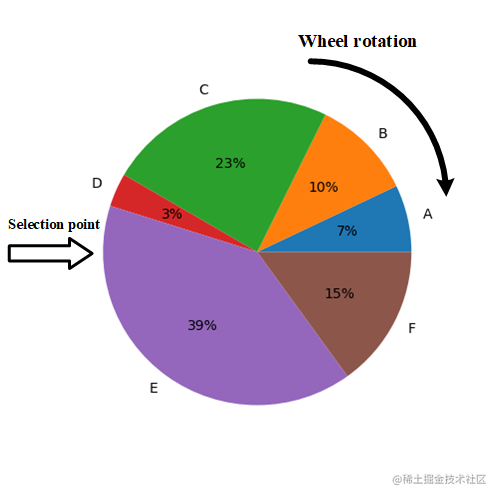### 随机遍历抽样（Stochastic universal sampling，SUS）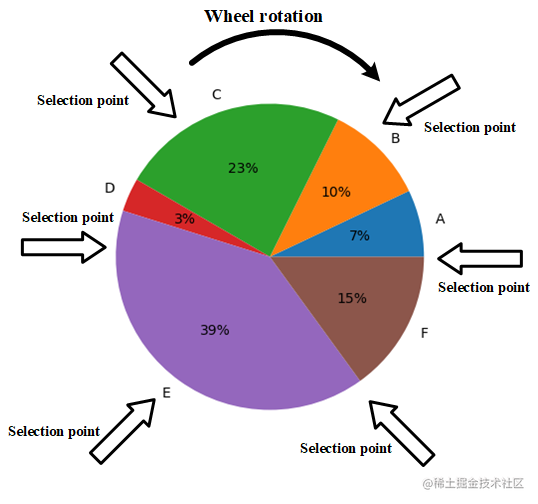### 排序选择（rank-based selection）

A829%
B12314%
C27523%
D414%
E45628%
F17419%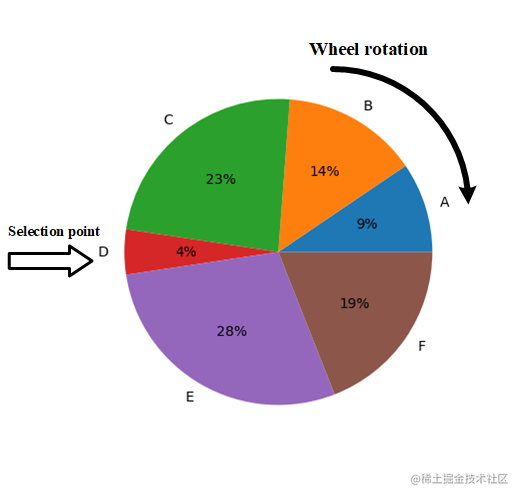### 适应度缩放选择(Fitness scaling)

$scaled fitness = a × (raw fitness) + b$

$50 = a × 4 + b \\ 100 = a × 45 + b$

$a = 1.22, b = 45.12$

$scaled fitness = 1.22 × (raw fitness) + 45.12$

A85012%
B126014%
C277819%
D45012%
E4510024%
F176616%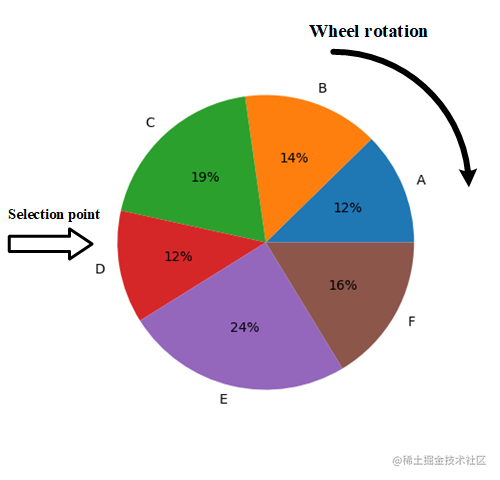### 锦标赛选择（Tournament selection）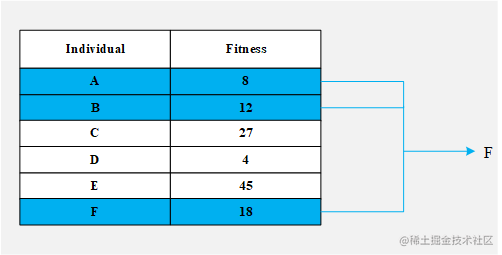## 常见交叉算子

### 单点交叉（Single-point crossover）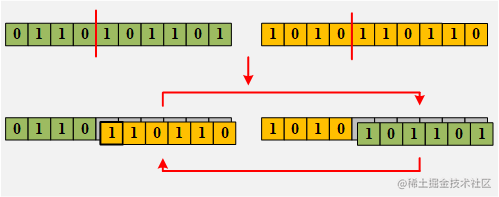### k点交叉（K-point crossover）

k点交叉是单点交叉的扩展，在交叉过程中使用k个交叉点，其中k表示正整数。 下图是k=2时的k点交叉示例，第一个交叉点位于第三和第四基因之间，另一个位于第七和第八个基因之间：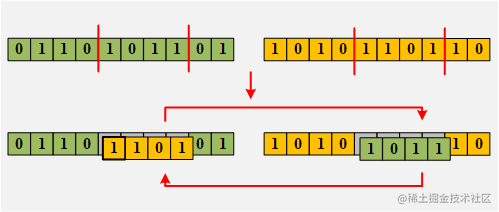### 均匀交叉（Uniform crossover）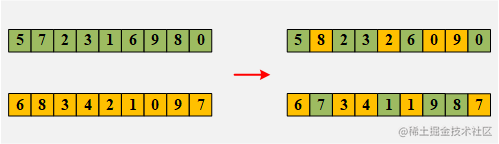Note: 在此示例中，第二个后代是通过对第一代后代的选择进行求补而创建的，但是，两个后代也可以彼此独立地创建。 由于这种方法不必交换染色体的全部片段，因此其后代具有更大的多样性。

### 用于有序列表的交叉

#### 有序交叉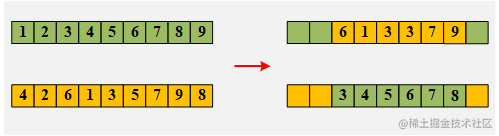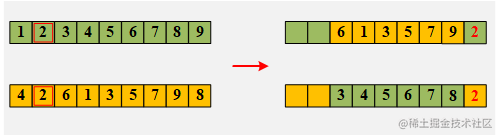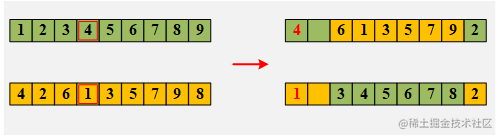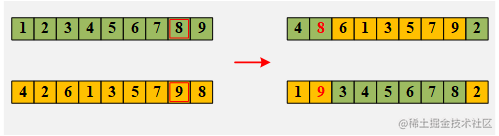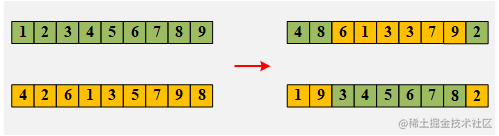## 常见变异算子

### 位翻转突变（Flip bit mutation）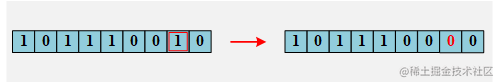### 交换突变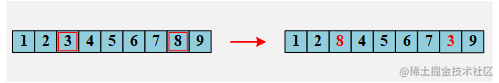### 反转突变（Swap mutation）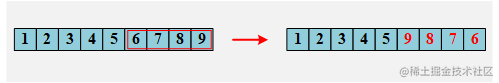### 倒换突变(Inversion mutation)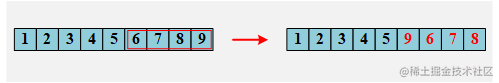## 常见用于实数编码染色体的遗传算子

1. 1.23和-30.10（第一维度）
2. 7.213和100.2（第二维度）
3. -25.39和42.42（第三维度）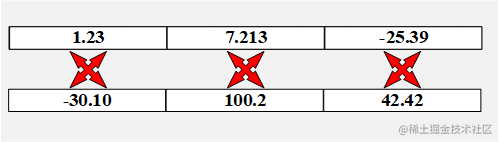### 混合交叉（blend crossover）

$[parent_1-α(parent_2-parent_1),parent_2+α(parent_2-parent_1)]$

1. α=0，将产生区间[1.33,5.72]
2. α=0.5，将产生区间[-0.865,7.915]
3. α=1.0，将产生区间[-3.06,10.11]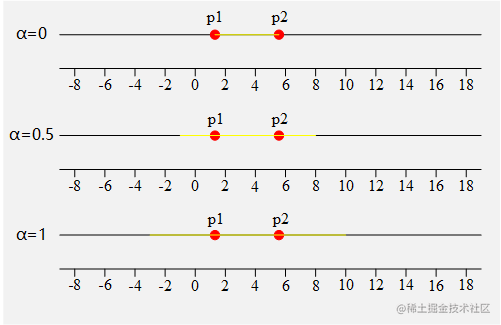### 模拟二进制交叉(simulated binary crossover)

$offspring_1=\frac 1 2 [(1+\beta)parent_1+(1-\beta)parent_2]$
$offspring_2=\frac 1 2 [(1-\beta)parent_1+(1+\beta)parent_2]$

1. 不管β的值如何，两个后代的平均值等于父母的平均值。
2. 当β值为1时，后代是双亲的副本。
3. 当β值小于1时，后代间的距离比亲代间的距离更短。
4. 当β值大于1时，后代间的距离比双亲间的距离更远。

1. β=0.8，产生后代1.769和5.281
2. β=1.0，产生后代1.33和5.72
3. β=1.2，产生后代0.891和6.159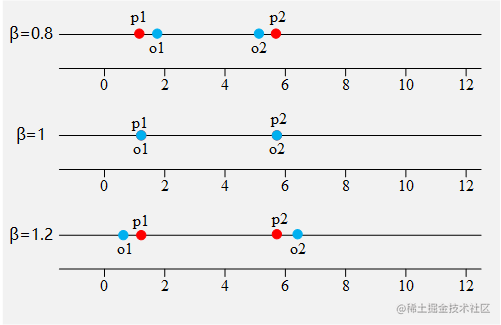$f(n) = \begin{cases} \beta=(2u)^{\frac {1} {\eta + 1} }, & \text{if n ≤ 0.5} \\ \beta=[\frac 1 {2(1-u)}]^{\frac {1} {\eta + 1} }, & \text{Otherwise} \end{cases}$

### 实数突变